# 推荐 ：手把手教你用Python创建简单的神经网络

+关注继续查看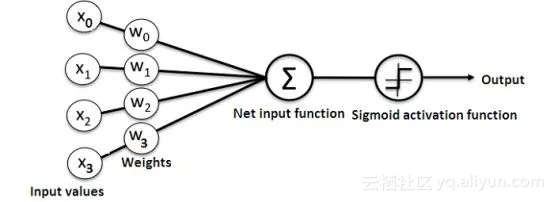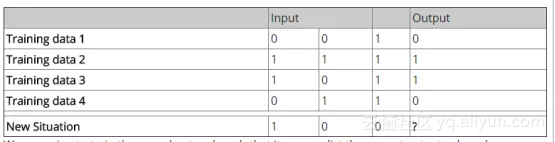numpy库提供了以下四种重要方法：

exp—用于生成自然指数

array—用于生成矩阵

dot—用于矩阵相乘

random—用于生成随机数。请注意，我们将生成随机数，以确保它们的有效分布。

1. 应用Sigmoid函数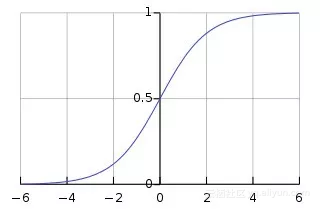1. 训练模型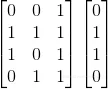import numpy as np

class NeuralNetwork():

def __init__(self):

# seeding for random number generation

np.random.seed(1)

#converting weights to a 3 by 1 matrix with values from -1 to 1 and mean of 0

self.synaptic_weights = 2 * np.random.random((3, 1)) - 1

def sigmoid(self, x):

#applying the sigmoid function

return 1 / (1 + np.exp(-x))

def sigmoid_derivative(self, x):

#computing derivative to the Sigmoid function

return x * (1 - x)

def train(self, training_inputs, training_outputs, training_iterations):

#training the model to make accurate predictions while adjusting weights continually

for iteration in range(training_iterations):

#siphon the training data via  the neuron

output = self.think(training_inputs)

#computing error rate for back-propagation

error = training_outputs - output

adjustments = np.dot(training_inputs.T, error * self.sigmoid_derivative(output))

def think(self, inputs):

#passing the inputs via the neuron to get output

#converting values to floats

inputs = inputs.astype(float)

output = self.sigmoid(np.dot(inputs, self.synaptic_weights))

return output

if __name__ == "__main__":

#initializing the neuron class

neural_network = NeuralNetwork()

print("Beginning Randomly Generated Weights: ")

print(neural_network.synaptic_weights)

#training data consisting of 4 examples--3 input values and 1 output

training_inputs = np.array([[0,0,1],

[1,1,1],

[1,0,1],

[0,1,1]])

training_outputs = np.array([[0,1,1,0]]).T

#training taking place

neural_network.train(training_inputs, training_outputs, 15000)

print("Ending Weights After Training: ")

print(neural_network.synaptic_weights)

user_input_one = str(input("User Input One: "))

user_input_two = str(input("User Input Two: "))

user_input_three = str(input("User Input Three: "))

print("Considering New Situation: ", user_input_one, user_input_two, user_input_three)

print("New Output data: ")

print(neural_network.think(np.array([user_input_one, user_input_two, user_input_three])))

print("Wow, we did it!")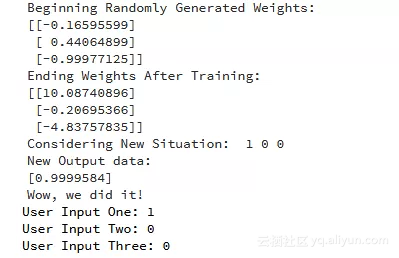ecshujufenxi”微信公众号6 04 月 23 日，首届 WAVE SUMMIT 2019 深度学习开发者峰会在北京举办，现场超千位开发者与来自百度、英特尔、清华大学等的科学家，就框架与深度学习展开了详细的讨论。在 WAVE 上，百度从建模、训练到部署，首次对外公布了 PaddlePaddle 全景图。
5 0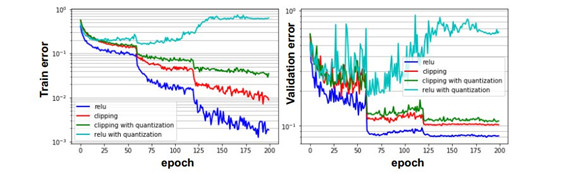SysML 2019论文解读：推理优化

5 04 月 16 日，北京智源人工智能研究院（Beijing Academy of Artificial Intelligence，BAAI，2018 年 11 月 14 日成立）在北京会议中心召开了「智源学者计划暨联合实验室发布会」。
4 0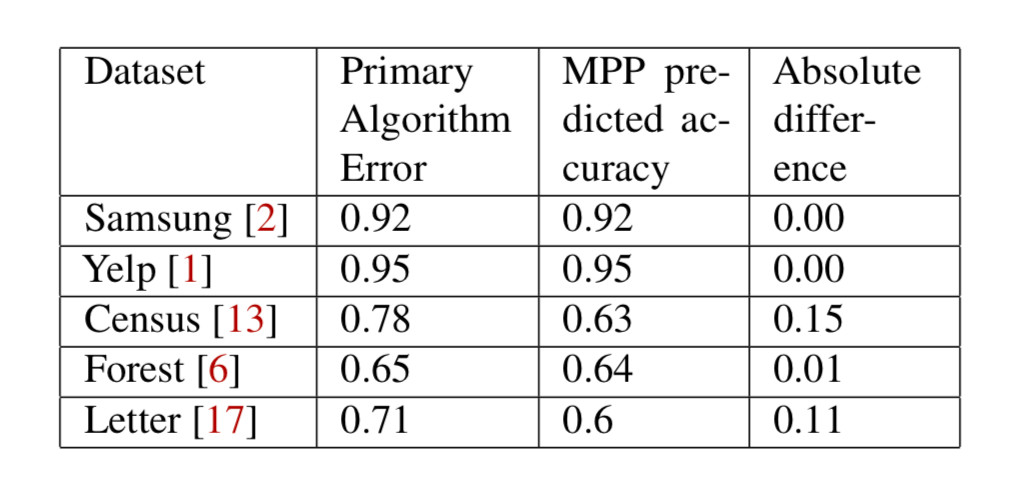OpML 2019提前看：模型表现预测与分布式机器学习
OpML 2019 全称是 2019 USENIX Conference on Operational Machine Learning，将于 2019 年 5 月 20 号在美国加州的 Santa Clara 举办。会议旨在提供科研人员和产业从业者一个交流合作的平台，为机器学习生产生命周期管理的普遍挑战开发并带来有影响力的研究进展和前沿解决方案。
3 0OpenAI 2:0击败Dota2 TI8冠军OG（鱼腩队？），「菜鸡」小编上手体验

6 03 0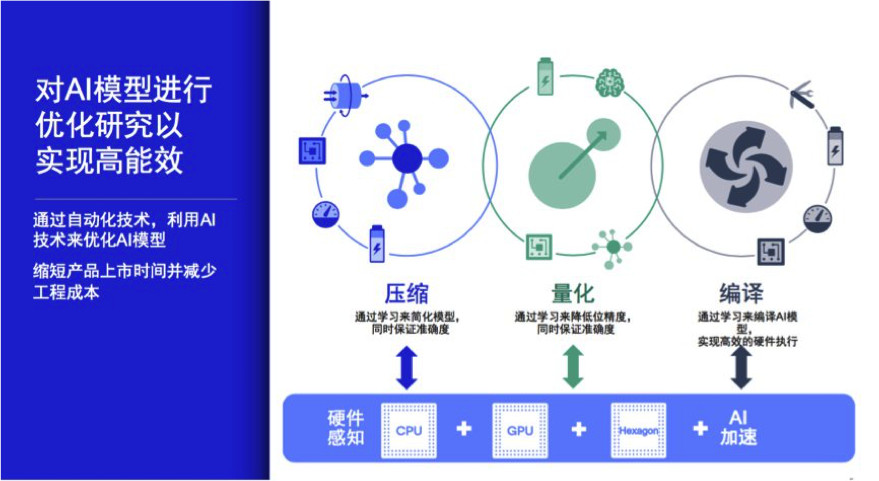3 0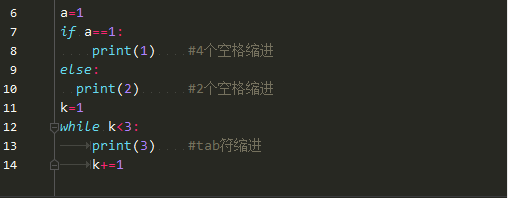python 对缩进是敏感的，而大多教程对缩进规则，往往就几句话带过，对于没有其他语言基础的初学者，十分不友好，本文就把python常见的缩进问题做了一些整理。
10 0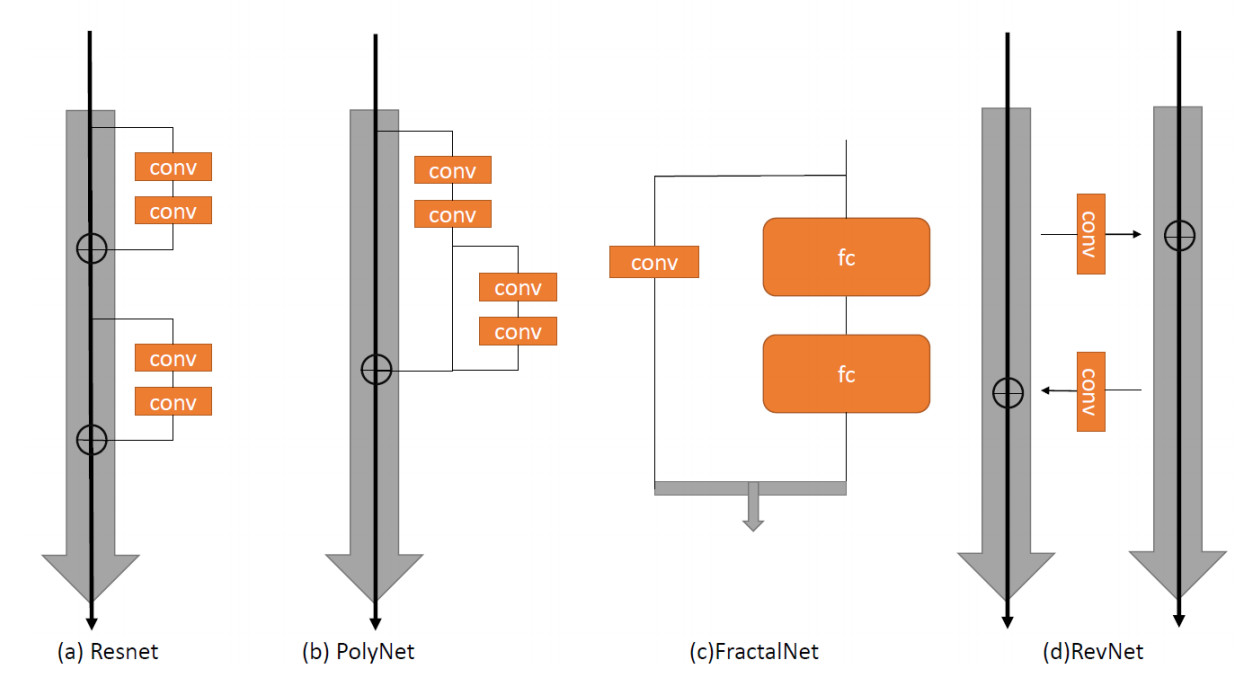3 0

5946

9

《SaaS模式云原生数据仓库应用场景实践》

《看见新力量：二》电子书